# 4.3 ggplot2简介

## 4.3.1  ggplot2和图形语法

ggplot2可以用来创建优雅的图形，由于它的灵活，简洁和一致的接口，可以提供美丽、可直接用来发表的图形，吸引了许多用户，特别是科研领域的用户。ggplot2使用grid包来提供一系列的高水平的函数，并将其延伸为图形语法，即独立指定绘图组件，并将它们组合起来，以构建我们想要的任何图形显示。图形语法包含6个主要成分：data, transformations, element, scales, guide和 coordinate system。图层图形语法源于多层数据构建图形的想法。它定义了下表中的图形组分：data, aesthetic mappings, statistical transformations, geometric objects, position adjustment, scales, coordinate system 和 faceting（数据、几何映射、统计变换、几何对象、位置调整、比例、坐标和面）。数据、几何映射、统计变换、几何对象、位置调整形成一个图层，一个图可以有多个图层。

• data 用于构造一个具体的图形，由变量组成，这些变量作为列存储在数据框中。数据独立于其他组件，可以应用多个数据集
• 映射：映射的目的是将数据属性（通常是数字或分类值）转换为几何或视觉属性；它用于指定几何属性的变量（例如，x位置、y位置、颜色、形状、大小等）
• Stat：转换数据，通常通过某种方式（例如，平滑线、回归线、装箱或聚合、箱线图、散点图等）对数据进行汇总。stat可以向数据集添加新变量。将几何映射到这些新变量是可能的
• 几何体：是指绘制来表示数据的几何对象；每个geom控制我们创建的打印类型。每个geom只能显示特定的几何图形（例如，条形图、线和点等），每个geom都有默认统计，并且每个统计都有默认的geom
• 位置调整：用于调整图形上几何元素的位置以避免相互遮挡，例如在条形图中，堆叠或回避（并排放置）条形以避免重叠。在散点图中，随机抖动点以减少过度绘制
• 尺度：每个几何属性都有一个函数，称为尺度；比例控制从数据到几何属性的映射，以确保数据值对该几何属性有效。此外，在统计变换之前执行缩放。
• 坐标：将对象的位置映射到绘图平面上。位置通常由两个坐标（x，y）指定，但可以是任意数量的坐标。此外，坐标变换发生在统计变换之后
• 面处理：在更一般的情节中称为条件图或网格图。面处理描述了应该使用哪些变量来分割数据，以及如何排列它们。刻面是一个强大的工具，可以研究不同的模式是否相同或不同于条件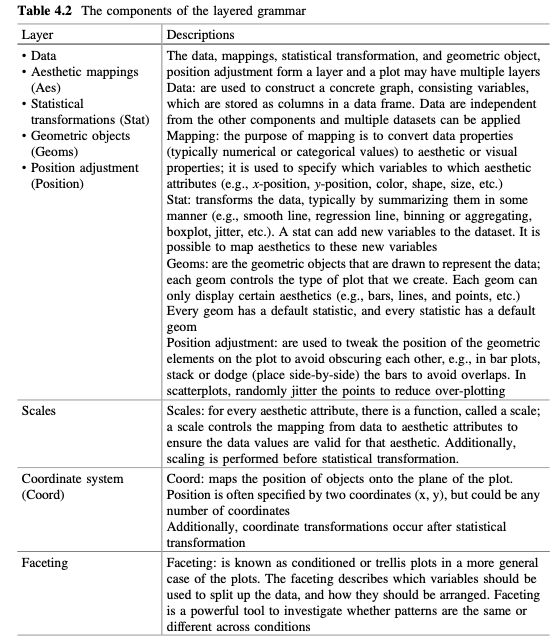图形语法的成分可以映射到分层语法的成分：分层语法的一层相当于图形语法的元素；分层语法的尺度相当于图形语法的尺度和指导；分层语法的坐标系和刻面等价于图形语法坐标系。然而，图形语法的翻译在ggplot2中没有对应关系（它的作用是由内置的R功能发挥的）。

## 4.3.2   使用gglot()创建绘图时的简单概念

Ggplot2的算法很简单：您提供数据，告诉ggplot2如何将变量映射到几何，使用什么图形，它负责细节。在ggplot2中，负责创建我们在绘图上感知到的对象。层由四个部分组成：数据几何映射、统计变换(STAT)、几何对象(GEOM)和位置调整(Wickham 2010)。一个图可能有多个图层。这些图层与坐标系和变换相结合，以生成最终的绘图。以下是一个情节生成过程：将变量映射到几何->分面数据->变换刻度->计算AESthetics->train scales->比例尺->渲染。

### 4.3.2.1 不用默认从头开始的全步骤

``library(ggplot2) ggplot() +  layer(    data = iris, mapping = aes(x = Sepal.Width, y = Sepal.Length),     geom = "point", stat = "identity", position = "identity"  )+ scale_y_continuous() +  scale_x_continuous()  + coord_cartesian()``

#### 4.3.2.2 用默认智能作图

• (1)每个geom都有一个默认的统计信息(反之亦然)，所以我们只需要指定geom或stat中的一个，而不是两个都指定。
• (2)不需要指定笛卡尔坐标系，因为它是默认坐标系
• (3)根据几何和变量类型增加默认比例。例如，对于位置，用线性比例变换连续值，并将分类值映射到整数；对于颜色，将连续变量映射到HCL颜色空间中的平滑路径，将离散变量映射到具有相等亮度和色度的均匀间隔的色调，例如，对于位置，连续值被映射到整数；对于颜色，连续变量被映射到HCL颜色空间中的平滑路径，离散变量被映射到具有相等亮度和色度的均匀分布的色调。因此，我们可以将上述代码减化如下：
``ggplot() +  layer(  data = iris, mapping = aes(x = Sepal.Width, y = Sepal.Length),  geom = "point"   )# 我这报错了，可能不同版本的区别？Error: Attempted to create layer with no stat.Run `rlang::last_error()` to see where the error occurred.``

``# 这就是我们常看到的代码的来历呀ggplot(iris, aes(Sepal.Width, Sepal.Length)) +  geom_point()``

``ggplot(iris, aes(Sepal.Width, Sepal.Length)) + geom_point() +stat_smooth(method = lm) +scale_x_log10() + scale_y_log10()``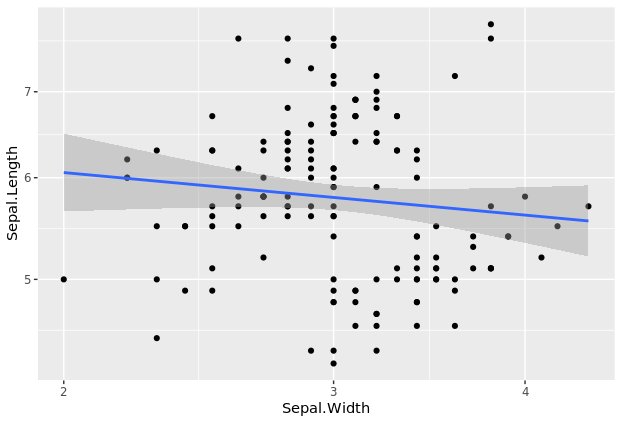如果不使用默认，代码将会如此冗长：

``# 发现这个代码也报错，可能被废弃了，或者我版本低？ggplot() +  layer(  data = iris, mapping = aes(x = Sepal.Width, y = Sepal.Length),  geom = "point", stat = "identity", position = "identity" )+  layer(    data = iris, mapping = aes(x = Sepal.Width, y = Sepal.Length), geom = "smooth", position = "identity",    stat = "smooth", method = lm)+  scale_y_log10() +  scale_x_log10() +  coord_cartesian()``

(Wickham 2010)论文中解释了默认优先级的概念。本书第5章中解释了如何逐层构建图。

### 4.3.2.3 通过使用qlot()减少键入语法代码的数量

``qplot(Sepal.Width, Sepal.Length, data = iris,  geom = c("point", "smooth"),       method = "lm", log = "xy")# 报了个警告`geom_smooth()` using formula 'y ~ x'Warning message:Ignoring unknown parameters: method ``

## 4.3.3 使用ggplot()绘图

### 4.3.3.1 创建一个层叠的图

ggplot2语法的第一个明显特性是分层，这意味着一个图至少由一个层创建，并通过使用gglot()函数向现有图添加更多玩家来增强。层结合了数据、美观映射、几何对象(Geom)、统计(统计变换)和位置调整。层由geom(几何对象)组成，因此ggplot2中的层也称为geom。因此，在ggplot2中，绘图实际上是由geom(例如geom_point())创建的，并由更多geom(例如geom_mooth()等)增强。一个几何图形表示一层地块。ggplot2的第二个显著特性是它使用数据帧，而不是单独的向量。因此，在使用该包创建绘图之前，如果数据是矢量，则需要将数据转换为数据帧。提供给gglot()本身或提供给各个geom以创建绘图的所有数据都包含在数据帧中。

• 第一步：为了初始化一个基本的gglot，我们从gglot()开始，创建一个包含数据和几何映射的Plot对象，我们将图形对象命名为p。
``# 这个花的数据我们应该想当熟悉了，这是我们最广泛使用的数据示例，来自R自带head(iris)#  Sepal.Length Sepal.Width Petal.Length Petal.Width Species1          5.1         3.5          1.4         0.2  setosa2          4.9         3.0          1.4         0.2  setosa3          4.7         3.2          1.3         0.2  setosa4          4.6         3.1          1.5         0.2  setosa5          5.0         3.6          1.4         0.2  setosa6          5.4         3.9          1.7         0.4  setosap <- ggplot(iris, aes(x=Sepal.Width, y=Sepal.Length))p``

``summary(p)# 150行，5列，就是上面的那个样式的数据# data: Sepal.Length, Sepal.Width, Petal.Length, Petal.Width,  Species [150x5]mapping:  x = ~Sepal.Width, y = ~Sepal.Lengthfaceting: <ggproto object: Class FacetNull, Facet, gg>    compute_layout: function``
• 第二步，使用几何图层geom_point()添加点，画一个散点图p 使用数据、几何映射和几何图形实现了ggplot2中的基本绘图。我们已经有了数据、美观地图的组件，需要添加的组件是散点图图层。可以使用**+运算符**添加图层，后跟定义带点散点图的函数：geom_point()
``p1 <- p + geom_point()p1summary(p1)# 前面一样，省略 -----------------------------------geom_point: na.rm = FALSEstat_identity: na.rm = FALSEposition_identity ``
• 第三步，为了绘制具有平滑曲线的散点图，我们在上一个plot对象中添加了一个名为geom_smooth()的附加几何层。对于这个图，我们将该方法设置为lm（线性模型的缩写）来绘制最佳拟合线
``# Add smoothing geom (layer2)p2 <- p1 + geom_smooth(method="lm")p2summary(p2)# data: Sepal.Length, Sepal.Width, Petal.Length, Petal.Width,  Species [150x5]mapping:  x = ~Sepal.Width, y = ~Sepal.Lengthfaceting: <ggproto object: Class FacetNull, Facet, gg>-----------------------------------geom_point: na.rm = FALSEstat_identity: na.rm = FALSEposition_identity geom_smooth: na.rm = FALSE, orientation = NA, se = TRUE, flipped_aes = FALSEstat_smooth: na.rm = FALSE, orientation = NA, se = TRUE, method = lmposition_identity``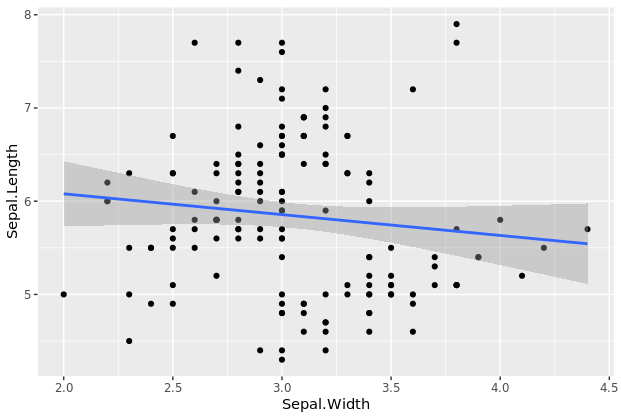输出显示，在plot对象中添加了一个附加层，该层使用线性模型进行拟合。曲线也有置信带，我们可以设置se=FALSE来关闭置信带。

``# 设置se=FALSE以关闭置信区间p1+geom_smooth(method=“lm”，se=FALSE)# `geom_smooth()` using formula 'y ~ x'``

### 4.3.3.2 使用比例来改变几何图层的美学效果

``p3 <- ggplot(iris, aes(x=Sepal.Width, y=Sepal.Length)) +   #Add scatterplot geom (layer1)   geom_point(col="blue", size=3) +   #Add smoothing geom (layer2)   geom_smooth(method="lm",col="red",size=2) p3``
``p4 <- ggplot(iris, aes(x=Sepal.Width, y=Sepal.Length)) +   #Add scatterplot geom (layer1)   geom_point(aes(col=Species), size=3) +   #Add smoothing geom (layer2)  geom_smooth(method="lm",col="red",size=2) p4``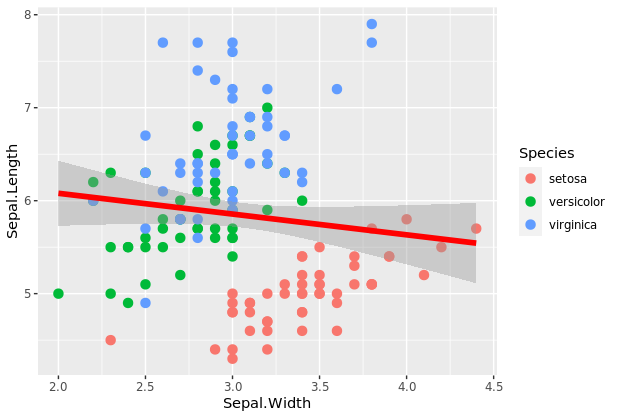我们可以看到，由于使用aes(col=Species)，散点图中的点根据其所属物种呈现不同的颜色。实际上，在ggplot2中，除了颜色之外，我们还可以使用大小、形状、笔划（边界的厚度）和填充（填充颜色）来区分适当绘图中的分组

### 4.3.3.3 使用坐标系统来调节和限制X轴和Y轴

``p5 <- p4 + coord_cartesian(xlim=c(2.2,4.2), ylim=c(4, 7)) # zooms in plot(p5)``

### 4.3.3.4 添加标签图层以更改标题和轴标签

``# 以下两图同样的效果p6 <- p5 + labs(title="Sepal width vs sepal length", subtitle="Using iris dataset", y="Length of Sepal", x="Width of Sepal")p6p7 <-p5 + ggtitle("Sepal width vs sepal length", subtitle="Using iris dataset") + ylab("Length of Sepal") + xlab("Width of Sepal")p7``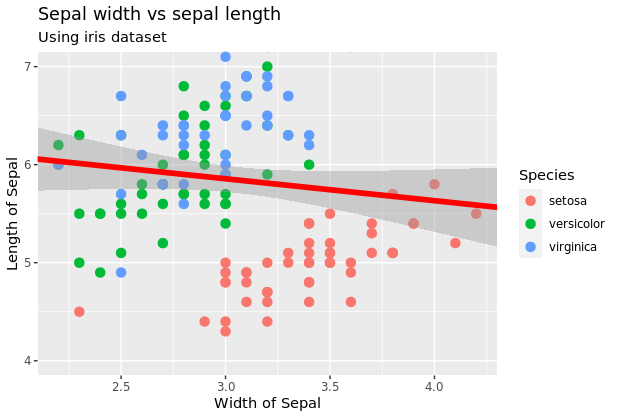到目前为止，代码整体如下；

``library(ggplot2)ggplot(iris, aes(x=Sepal.Width, y=Sepal.Length)) +  geom_point(aes(col=Species), size=3) +  geom_smooth(method="lm",col="red",size=2) +  coord_cartesian(xlim=c(2.2,4.2), ylim=c(4, 7)) +  labs(title="Sepal width vs sepal length", subtitle="Using iris dataset",         y="Length of Sepal", x="Width of Sepal")``

### 4.3.3.5 使用刻面检测不同条件下的模式

#### 使用facet_grid(公式)在栅格中绘制多个图

``#Spliting plots by rowsggplot(iris, aes(x=Sepal.Width, y=Sepal.Length)) +    geom_point(aes(col=Species), size=3) +   geom_smooth(method="lm",col="red",size=2) +   coord_cartesian(xlim=c(2.2,4.2), ylim=c(4, 7)) +   # Add Facet Grid   facet_grid(Species ~.)``
``#Spliting plots by columns  ggplot(iris, aes(x=Sepal.Width, y=Sepal.Length))  +   geom_point(aes(col=Species), size=3) +   geom_smooth(method="lm",col="red",size=2) +  coord_cartesian(xlim=c(2.2,4.2), ylim=c(4, 7)) +  # Add Facet Grid   facet_grid(.~ Species)``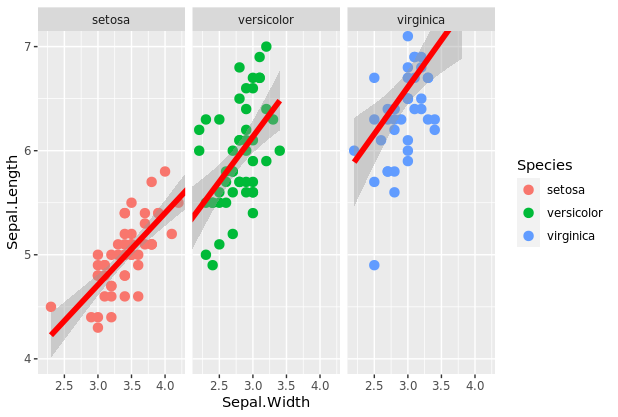如果添加margin = TRUE的选项，会多一个所有数据的图。

`` ggplot(iris, aes(x=Sepal.Width, y=Sepal.Length))  +    geom_point(aes(col=Species), size=3) +   geom_smooth(method="lm",col="red",size=2) +   coord_cartesian(xlim=c(2.2,4.2), ylim=c(4, 7)) +   # Add Facet Grid   facet_grid(.~ Species, margin=TRUE)``如果我们想要基于两个或更多变量来分割曲线图，我们需要对所有这些变量执行刻面。例如，公式.~y+z(facet_grid(.~y+z))对两个变量执行刻面，两个变量都按列显示，绘图将基于一个变量与另一个变量的级别并排显示。这种可视化使得两个分类变量的比较非常有效。在这个公式中，我们可以看到使用**+运算符**将附加变量z加到y上。

#### 使用facet_wrap(公式)将一大系列绘图分解为多个小绘图

wrap刻面将一系列大绘图生成单个类别的多个小绘图。此功能使包装分面特别适用于对多个级别的类别变量的分面组合进行分面。要执行WRAP刻面，我们使用facet_wrap(FORMULA)函数。刻面变量可以以参数的形式列出，形式为Facet_wrap(x~y+z)。~符号左边的变量形成行，而右边的变量形成列。Facet_wrap(x~.)的语法。用于在行中仅按x拆分绘图，并包括绘图中的所有其他子集。与前面一个函数的区别是，facet_wrap(FORMULA)可以选择网格中的行数和列数。我们可以分别使用nrow和ncol参数指定它们。

`` #Facet Wrap #Splitting plots by columnsggplot(iris, aes(x=Sepal.Width, y=Sepal.Length)) +   geom_point(aes(col=Species), size=3) +  geom_smooth(method="lm",col="red",size=2) +  coord_cartesian(xlim=c(2.2,4.2), ylim=c(4, 7)) +  #Add Facet Wrap  facet_wrap(~ Species, nrow=2)``